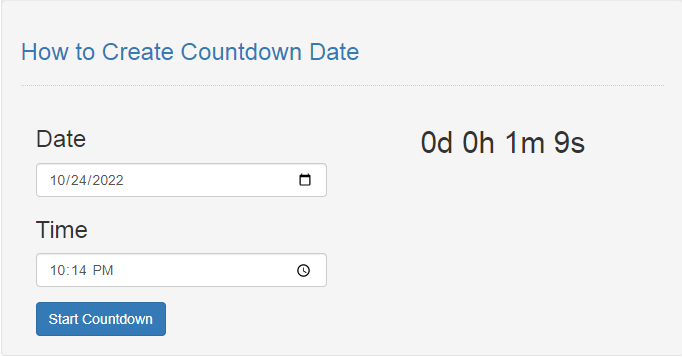# How to Create Countdown Date in JavaScript

## Introduction

In this tutorial we will create a How to Create Countdown Date in JavaScript. This tutorial purpose is to provide you a technique to do the countdown date. This will tackle the applying of a countdown timer for a strong>DateTime value. I will provide a sample program to show the actual coding of this tutorial.

This tutorial is simple and easy to understand just follow the instruction I provided and you will do it without a problem. This program is useful if you to apply a timer for some event in your system. I will try my best to give you the easiest way of creating this program Countdown Date Timer. So let's do the coding.

## Before we get started:

This is the link for the template that i used for the layout design https://getbootstrap.com/.

## Creating The Interface

This is where we will create a simple interface for our application. This code will display the form for the date time entry. To create this simply copy and write it into your text editor, then save it as index.html.
`<!DOCTYPE html><html lang="en">	<head>		<meta charset="UTF-8" name="viewport" content="width=device-width, initial-scale=1"/>		<link rel="stylesheet" type="text/css" href="css/bootstrap.css"/>	</head><body>	<nav class="navbar navbar-default">		<div class="container-fluid">			<a class="navbar-brand" href="https://sourcecodester.com">Sourcecodester</a>		</div>	</nav>	<div class="col-md-3"></div>	<div class="col-md-6 well">		<h3 class="text-primary">How to Create Countdown Date</h3>		<hr style="border-top:1px dotted #ccc;"/>		<div class="col-md-6">			<div class="form-group">				<h3>Date</h3>				<input type="date" id="date" class="form-control"/>			</div>			<div class="form-group">				<h3>Time</h3>				<input type="time" id="time" class="form-control"/>			</div>			<button type="button" class="btn btn-primary" onclick="setCount()">Start Countdown</button>		</div>		<div class="col-md-6">		<center><h2 id="display">00:00:00</h2></center>		</div>  	</div></body><script src="script.js"></script></html>`

## Creating JavaScript Function

This is where the main function of the application is. This code will start the timer when the inputs is already entered. To do this just copy and write these block of codes inside the text editor and save it as script.js.
`function setCount(){	var date = document.getElementById('date');	var time = document.getElementById('time'); 	if(date.value == "" || time.value == ""){		alert("Please complete the required field!");	}else{		var countDate = new Date(date.value+" "+time.value).getTime(); 		var countDay = new Date(date.value+" "+time.value).getDate(); 		var x = setInterval(function(){			var nowTime = new Date().getTime();			var nowDate = new Date().getDate();			var distance = countDate - nowTime;			var dayDis = countDay - nowDate; 			var hours = Math.floor((distance % (1000 * 60 * 60 * 24)) / (1000 * 60 * 60));			var minutes = Math.floor((distance % (1000 * 60 * 60)) / (1000 * 60));			var seconds = Math.floor((distance % (1000 * 60)) / 1000); 			document.getElementById("display").innerHTML = dayDis + "d " + hours + "h "			+ minutes + "m " + seconds + "s ";  			if (distance < 0) {				clearInterval(x);				document.getElementById("display").innerHTML = "Time Expired";			} 		}, 1000);	}}`

In this code we display first the form for our Date Time value. We bind the form field with id so that it will be easier to fetch the date. After that we create a new variable called nowTime and nowDay. We computed the distance by the difference of countDate and nowTime, and also dayDis the value is the difference of countDay and nowDate. After we set our variables we finally calculated the hours, date, and seconds using the Math.floor() function.

## Output: